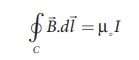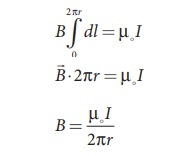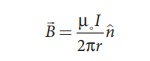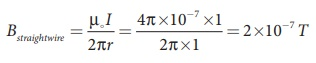Home | | Physics 12th Std | Magnetic field due to the current carrying wire of infinite length using Ampere’s law

# Magnetic field due to the current carrying wire of infinite length using Ampere’s law

Ampere’s Circuital Law | Physics: Magnetic field due to the current carrying wire of infinite length using Ampere’s law

Magnetic field due to the current carrying wire of infinite length using Ampère’s lawConsider a straight conductor of infinite length carrying current I and the direction ofmagnetic field lines is shown in Figure 3.42. Since the wire is geometrically cylindrical in shape and symmetrical about its axis, we construct an Ampèrian loop in the form of a circular shape at a distance r from the centre of the conductor as shown in Figure 3.42. From the Ampère’s law, we getwhereis the line element along the amperian loop (tangent to the circular loop). Hence, the angle between magnetic field vector and line element is zero. Therefore,where I is the current enclosed by the Ampèrian loop. Due to the symmetry, the magnitude of the magnetic field is uniform over the Ampèrian loop, we can take B out of the integration.For a circular loop, the circumference is 2πr, which implies,In vector form, the magnetic field iswhere n is the unit vector along the tangent to the Ampèrian loop as shown in the Figure 3.42. This perfectly agrees with the result obtained from Biot-Savart’s law as given in equation (3.39).

EXAMPLE 3.18

Compute the magnitude of the magnetic field of a long, straight wire carrying a current of 1A at distance of 1m from it. Compare it with Earth’s magnetic field.

Solution

Given that 1 = 1 A and radius r = 1 mBut the Earth’s magnetic field is BEarth  ≈ 10−5 T

So, Bstraightwire is one hundred times smaller than BEarth.

Tags : Ampere’s Circuital Law | Physics , 12th Physics : Magnetism and Magnetic Effects of Electric Current
Study Material, Lecturing Notes, Assignment, Reference, Wiki description explanation, brief detail
12th Physics : Magnetism and Magnetic Effects of Electric Current : Magnetic field due to the current carrying wire of infinite length using Ampere’s law | Ampere’s Circuital Law | Physics